Auto ByteScience AI# ESRGAN：基于GAN的增强超分辨率方法（附代码解析）

ESRGAN: Enhanced Super-Resolution Generative Adversarial Networks 发表于 ECCV 2018 的 Workshops，作者在 SRGAN 的基础上进行了改进，包括改进网络的结构、判决器的判决形式，以及更换了一个用于计算感知域损失的预训练网络。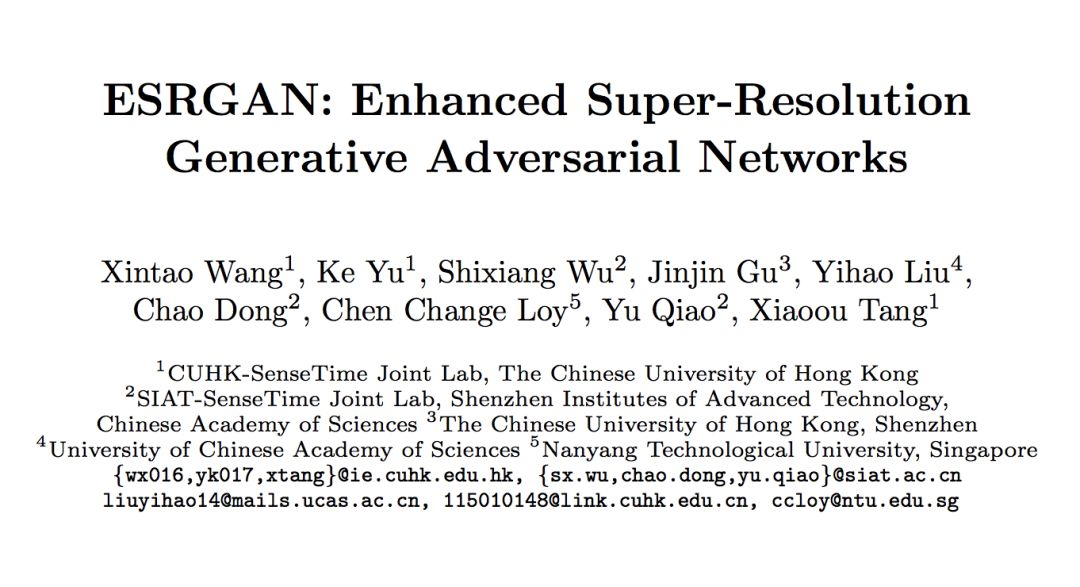超分辨率生成对抗网络（SRGAN）是一项开创性的工作，能够在单一图像超分辨率中生成逼真的纹理。这项工作发表于 CVPR 2017。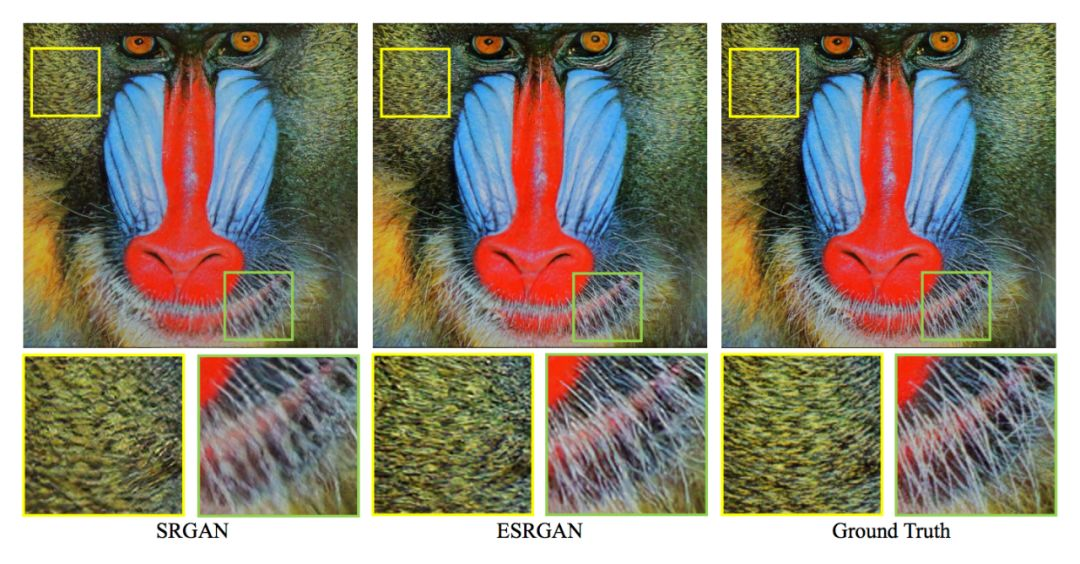在纹理和细节上，ESRGAN都优于SRGAN

### ESRGAN的改进

1. 网络的基本单元从基本的残差单元变为 Residual-in-Residual Dense Block (RRDB)；

2. GAN 网络改进为 Relativistic average GAN (RaGAN)；

3. 改进感知损失函数，使用激活前的 VGG 特征，这个改进会提供更尖锐的边缘和更符合视觉的结果。

### 网络结构及思想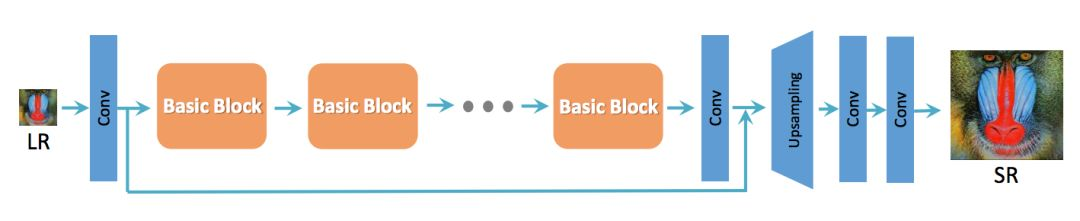SRResNet基本结构

1. 去掉所有的 BN 层；

2. 把原始的 block 变为 Residual-in-Residual Dense Block (RRDB)，这个 block 结合了多层的残差网络和密集连接。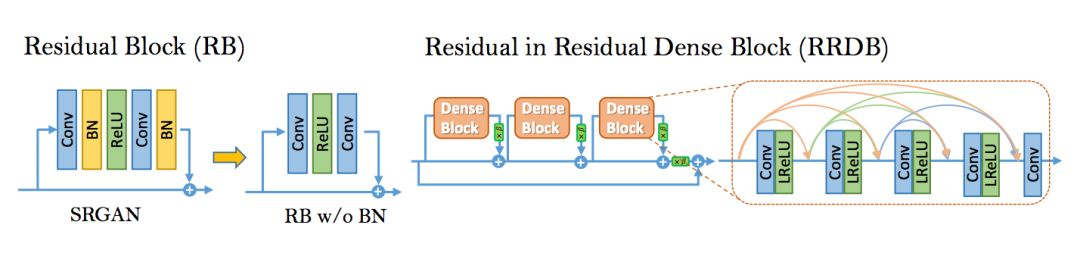思想

BN 层的影响：对于不同的基于 PSNR 的任务（包括超分辨率和去模糊）来说，去掉 BN 层已经被证明会提高表现和减小计算复杂度。

BN 层在训练时，使用一个 batch 的数据的均值和方差对该 batch 特征进行归一化，在测试时，使用在整个测试集上的数据预测的均值和方差。当训练集和测试集的统计量有很大不同的时候，BN 层就会倾向于生成不好的伪影，并且限制模型的泛化能力。

Trick

1. 对残差信息进行 scaling，即将残差信息乘以一个 0 到 1 之间的数，用于防止不稳定；

2. 更小的初始化，作者发现当初始化参数的方差变小时，残差结构更容易进行训练。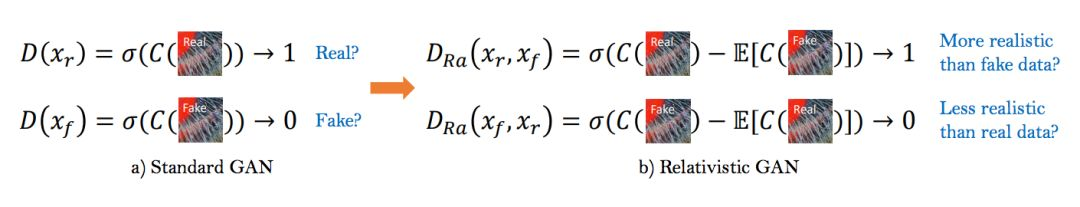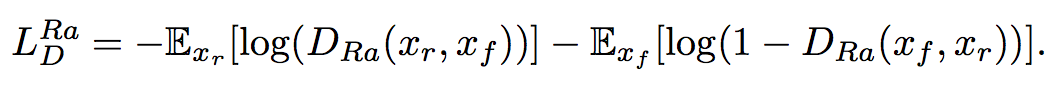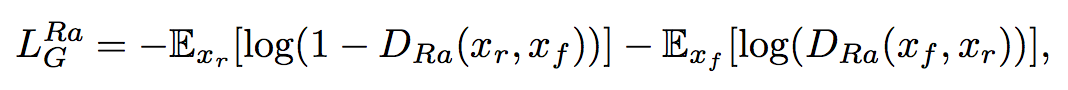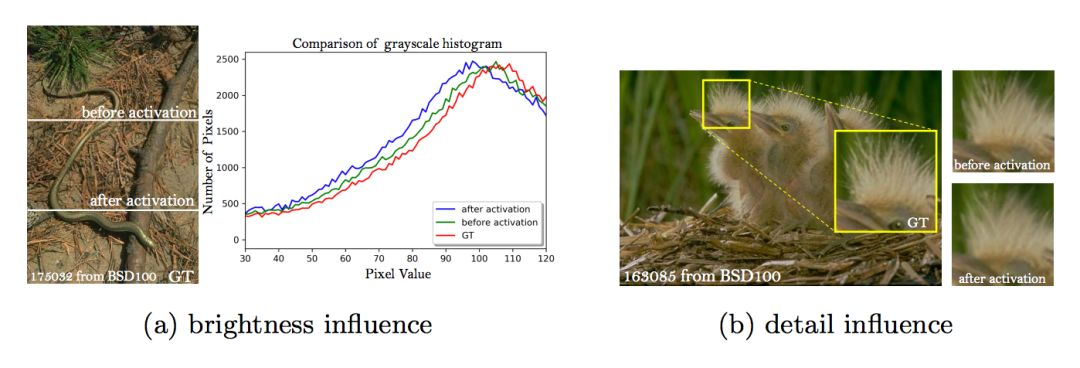使用激活前与激活后的特征的比较：a. 亮度 b. 细节

### 损失函数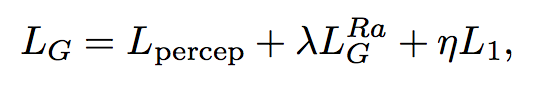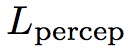即为感知域损失，作者的源码取的是 L1 Loss，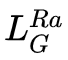即为上面定义的生成器损失，而 L1 则为 pixel-wise 损失，即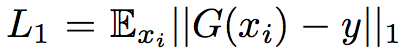, 实验中取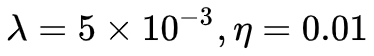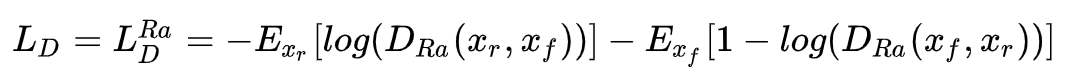### 网络细节

``````def conv_block(in_nc, out_nc, kernel_size, stride=1, dilation=1, groups=1, bias=True, \
pad_type='zero', norm_type=None, act_type='relu', mode='CNA'):
'''
Conv layer with padding, normalization, activation
mode: CNA --> Conv -> Norm -> Act
NAC --> Norm -> Act --> Conv (Identity Mappings in Deep Residual Networks, ECCV16)
'''
assert mode in ['CNA', 'NAC', 'CNAC'], 'Wong conv mode [{:s}]'.format(mode)

c = nn.Conv2d(in_nc, out_nc, kernel_size=kernel_size, stride=stride, padding=padding, \
dilation=dilation, bias=bias, groups=groups)
a = act(act_type) if act_type else None
if 'CNA' in mode:
n = norm(norm_type, out_nc) if norm_type else None
return sequential(p, c, n, a)
elif mode == 'NAC':
if norm_type is None and act_type is not None:
a = act(act_type, inplace=False)
# Important!
# input----ReLU(inplace)----Conv--+----output
#        |________________________|
# inplace ReLU will modify the input, therefore wrong output
n = norm(norm_type, in_nc) if norm_type else None
return sequential(n, a, p, c)
``````

``````def pad(pad_type, padding):
# helper selecting padding layer
# if padding is 'zero', do by conv layers
if padding == 0:
return None
if pad_type == 'reflect':
elif pad_type == 'replicate':
else:
raise NotImplementedError('padding layer [{:s}] is not implemented'.format(pad_type))
return layer

kernel_size = kernel_size + (kernel_size - 1) * (dilation - 1)
padding = (kernel_size - 1) // 2
``````

``````class ResidualDenseBlock_5C(nn.Module):
'''
Residual Dense Block
style: 5 convs
The core module of paper: (Residual Dense Network for Image Super-Resolution, CVPR 18)
'''

def __init__(self, nc, kernel_size=3, gc=32, stride=1, bias=True, pad_type='zero', \
norm_type=None, act_type='leakyrelu', mode='CNA'):
super(ResidualDenseBlock_5C, self).__init__()
# gc: growth channel, i.e. intermediate channels
self.conv1 = conv_block(nc, gc, kernel_size, stride, bias=bias, pad_type=pad_type, \
norm_type=norm_type, act_type=act_type, mode=mode)
self.conv2 = conv_block(nc+gc, gc, kernel_size, stride, bias=bias, pad_type=pad_type, \
norm_type=norm_type, act_type=act_type, mode=mode)
self.conv3 = conv_block(nc+2*gc, gc, kernel_size, stride, bias=bias, pad_type=pad_type, \
norm_type=norm_type, act_type=act_type, mode=mode)
self.conv4 = conv_block(nc+3*gc, gc, kernel_size, stride, bias=bias, pad_type=pad_type, \
norm_type=norm_type, act_type=act_type, mode=mode)
if mode == 'CNA':
last_act = None
else:
last_act = act_type
self.conv5 = conv_block(nc+4*gc, nc, 3, stride, bias=bias, pad_type=pad_type, \
norm_type=norm_type, act_type=last_act, mode=mode)

def forward(self, x):
x1 = self.conv1(x)
x2 = self.conv2(torch.cat((x, x1), 1))
x3 = self.conv3(torch.cat((x, x1, x2), 1))
x4 = self.conv4(torch.cat((x, x1, x2, x3), 1))
x5 = self.conv5(torch.cat((x, x1, x2, x3, x4), 1))
return x5.mul(0.2) + x
``````

``````class RRDB(nn.Module):
'''
Residual in Residual Dense Block
(ESRGAN: Enhanced Super-Resolution Generative Adversarial Networks)
'''

def __init__(self, nc, kernel_size=3, gc=32, stride=1, bias=True, pad_type='zero', \
norm_type=None, act_type='leakyrelu', mode='CNA'):
super(RRDB, self).__init__()
self.RDB1 = ResidualDenseBlock_5C(nc, kernel_size, gc, stride, bias, pad_type, \
norm_type, act_type, mode)
self.RDB2 = ResidualDenseBlock_5C(nc, kernel_size, gc, stride, bias, pad_type, \
norm_type, act_type, mode)
self.RDB3 = ResidualDenseBlock_5C(nc, kernel_size, gc, stride, bias, pad_type, \
norm_type, act_type, mode)

def forward(self, x):
out = self.RDB1(x)
out = self.RDB2(out)
out = self.RDB3(out)
return out.mul(0.2) + x
``````

``````def upconv_blcok(in_nc, out_nc, upscale_factor=2, kernel_size=3, stride=1, bias=True, \
pad_type='zero', norm_type=None, act_type='relu', mode='nearest'):
# Up conv
# described in https://distill.pub/2016/deconv-checkerboard/
upsample = nn.Upsample(scale_factor=upscale_factor, mode=mode)
conv = conv_block(in_nc, out_nc, kernel_size, stride, bias=bias, \
return sequential(upsample, conv)
``````

``````class ShortcutBlock(nn.Module):
#Elementwise sum the output of a submodule to its input
def __init__(self, submodule):
super(ShortcutBlock, self).__init__()
self.sub = submodule

def forward(self, x):
output = x + self.sub(x)
return output

def __repr__(self):
tmpstr = 'Identity + \n|'
modstr = self.sub.__repr__().replace('\n', '\n|')
tmpstr = tmpstr + modstr
return tmpstr
``````

``````class RRDBNet(nn.Module):
def __init__(self, in_nc, out_nc, nf, nb, gc=32, upscale=4, norm_type=None, \
act_type='leakyrelu', mode='CNA', upsample_mode='upconv'):
super(RRDBNet, self).__init__()
n_upscale = int(math.log(upscale, 2))
if upscale == 3:
n_upscale = 1

fea_conv = B.conv_block(in_nc, nf, kernel_size=3, norm_type=None, act_type=None)
rb_blocks = [B.RRDB(nf, kernel_size=3, gc=32, stride=1, bias=True, pad_type='zero', \
norm_type=norm_type, act_type=act_type, mode='CNA') for _ in range(nb)]
LR_conv = B.conv_block(nf, nf, kernel_size=3, norm_type=norm_type, act_type=None, mode=mode)

if upsample_mode == 'upconv':
upsample_block = B.upconv_blcok
elif upsample_mode == 'pixelshuffle':
upsample_block = B.pixelshuffle_block
else:
raise NotImplementedError('upsample mode [{:s}] is not found'.format(upsample_mode))
if upscale == 3:
upsampler = upsample_block(nf, nf, 3, act_type=act_type)
else:
upsampler = [upsample_block(nf, nf, act_type=act_type) for _ in range(n_upscale)]
HR_conv0 = B.conv_block(nf, nf, kernel_size=3, norm_type=None, act_type=act_type)
HR_conv1 = B.conv_block(nf, out_nc, kernel_size=3, norm_type=None, act_type=None)

self.model = B.sequential(fea_conv, B.ShortcutBlock(B.sequential(*rb_blocks, LR_conv)),\
*upsampler, HR_conv0, HR_conv1)

def forward(self, x):
x = self.model(x)
return x
``````

``````# VGG style Discriminator with input size 128*128
class Discriminator_VGG_128(nn.Module):
def __init__(self, in_nc, base_nf, norm_type='batch', act_type='leakyrelu', mode='CNA'):
super(Discriminator_VGG_128, self).__init__()
# features
# hxw, c
# 128, 64
conv0 = B.conv_block(in_nc, base_nf, kernel_size=3, norm_type=None, act_type=act_type, \
mode=mode)
conv1 = B.conv_block(base_nf, base_nf, kernel_size=4, stride=2, norm_type=norm_type, \
act_type=act_type, mode=mode)
# 64, 64
conv2 = B.conv_block(base_nf, base_nf*2, kernel_size=3, stride=1, norm_type=norm_type, \
act_type=act_type, mode=mode)
conv3 = B.conv_block(base_nf*2, base_nf*2, kernel_size=4, stride=2, norm_type=norm_type, \
act_type=act_type, mode=mode)
# 32, 128
conv4 = B.conv_block(base_nf*2, base_nf*4, kernel_size=3, stride=1, norm_type=norm_type, \
act_type=act_type, mode=mode)
conv5 = B.conv_block(base_nf*4, base_nf*4, kernel_size=4, stride=2, norm_type=norm_type, \
act_type=act_type, mode=mode)
# 16, 256
conv6 = B.conv_block(base_nf*4, base_nf*8, kernel_size=3, stride=1, norm_type=norm_type, \
act_type=act_type, mode=mode)
conv7 = B.conv_block(base_nf*8, base_nf*8, kernel_size=4, stride=2, norm_type=norm_type, \
act_type=act_type, mode=mode)
# 8, 512
conv8 = B.conv_block(base_nf*8, base_nf*8, kernel_size=3, stride=1, norm_type=norm_type, \
act_type=act_type, mode=mode)
conv9 = B.conv_block(base_nf*8, base_nf*8, kernel_size=4, stride=2, norm_type=norm_type, \
act_type=act_type, mode=mode)
# 4, 512
self.features = B.sequential(conv0, conv1, conv2, conv3, conv4, conv5, conv6, conv7, conv8,\
conv9)

# classifier
self.classifier = nn.Sequential(
nn.Linear(512 * 4 * 4, 100), nn.LeakyReLU(0.2, True), nn.Linear(100, 1))

def forward(self, x):
x = self.features(x)
x = x.view(x.size(0), -1)
x = self.classifier(x)
return x
``````

``````class MINCNet(nn.Module):
def __init__(self):
super(MINCNet, self).__init__()
self.ReLU = nn.ReLU(True)
self.conv11 = nn.Conv2d(3, 64, 3, 1, 1)
self.conv12 = nn.Conv2d(64, 64, 3, 1, 1)
self.maxpool1 = nn.MaxPool2d(2, stride=2, padding=0, ceil_mode=True)
self.conv21 = nn.Conv2d(64, 128, 3, 1, 1)
self.conv22 = nn.Conv2d(128, 128, 3, 1, 1)
self.maxpool2 = nn.MaxPool2d(2, stride=2, padding=0, ceil_mode=True)
self.conv31 = nn.Conv2d(128, 256, 3, 1, 1)
self.conv32 = nn.Conv2d(256, 256, 3, 1, 1)
self.conv33 = nn.Conv2d(256, 256, 3, 1, 1)
self.maxpool3 = nn.MaxPool2d(2, stride=2, padding=0, ceil_mode=True)
self.conv41 = nn.Conv2d(256, 512, 3, 1, 1)
self.conv42 = nn.Conv2d(512, 512, 3, 1, 1)
self.conv43 = nn.Conv2d(512, 512, 3, 1, 1)
self.maxpool4 = nn.MaxPool2d(2, stride=2, padding=0, ceil_mode=True)
self.conv51 = nn.Conv2d(512, 512, 3, 1, 1)
self.conv52 = nn.Conv2d(512, 512, 3, 1, 1)
self.conv53 = nn.Conv2d(512, 512, 3, 1, 1)

def forward(self, x):
out = self.ReLU(self.conv11(x))
out = self.ReLU(self.conv12(out))
out = self.maxpool1(out)
out = self.ReLU(self.conv21(out))
out = self.ReLU(self.conv22(out))
out = self.maxpool2(out)
out = self.ReLU(self.conv31(out))
out = self.ReLU(self.conv32(out))
out = self.ReLU(self.conv33(out))
out = self.maxpool3(out)
out = self.ReLU(self.conv41(out))
out = self.ReLU(self.conv42(out))
out = self.ReLU(self.conv43(out))
out = self.maxpool4(out)
out = self.ReLU(self.conv51(out))
out = self.ReLU(self.conv52(out))
out = self.conv53(out)
return out
``````

``````class MINCFeatureExtractor(nn.Module):
def __init__(self, feature_layer=34, use_bn=False, use_input_norm=True, \
device=torch.device('cpu')):
super(MINCFeatureExtractor, self).__init__()

self.features = MINCNet()
self.features.eval()
# No need to BP to variable
for k, v in self.features.named_parameters():

def forward(self, x):
output = self.features(x)
return output
``````

### 网络插值思想### 训练细节

HR patch 大小：128×128。实验发现使用大的 patch 时，训练一个深层网络效果会更好，因为一个增大的感受域会帮助模型捕捉更具有语义的信息。

1. 训练一个基于 PSNR 指标的模型（L1 Loss），初始化学习率：2×1e-4，每 200000 个 mini-batch 学习率除以 2；

2. 以 1 中训练的模型作为生成器的初始化。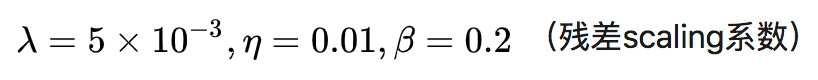1. 可以避免生成器不希望的局部最优；

2. 再预训练以后，判别器所得到的输入图像的质量是相对较好的，而不是完全初始化的图像，这样会使判别器更关注到纹理的判别。

### 对比实验（4倍放大）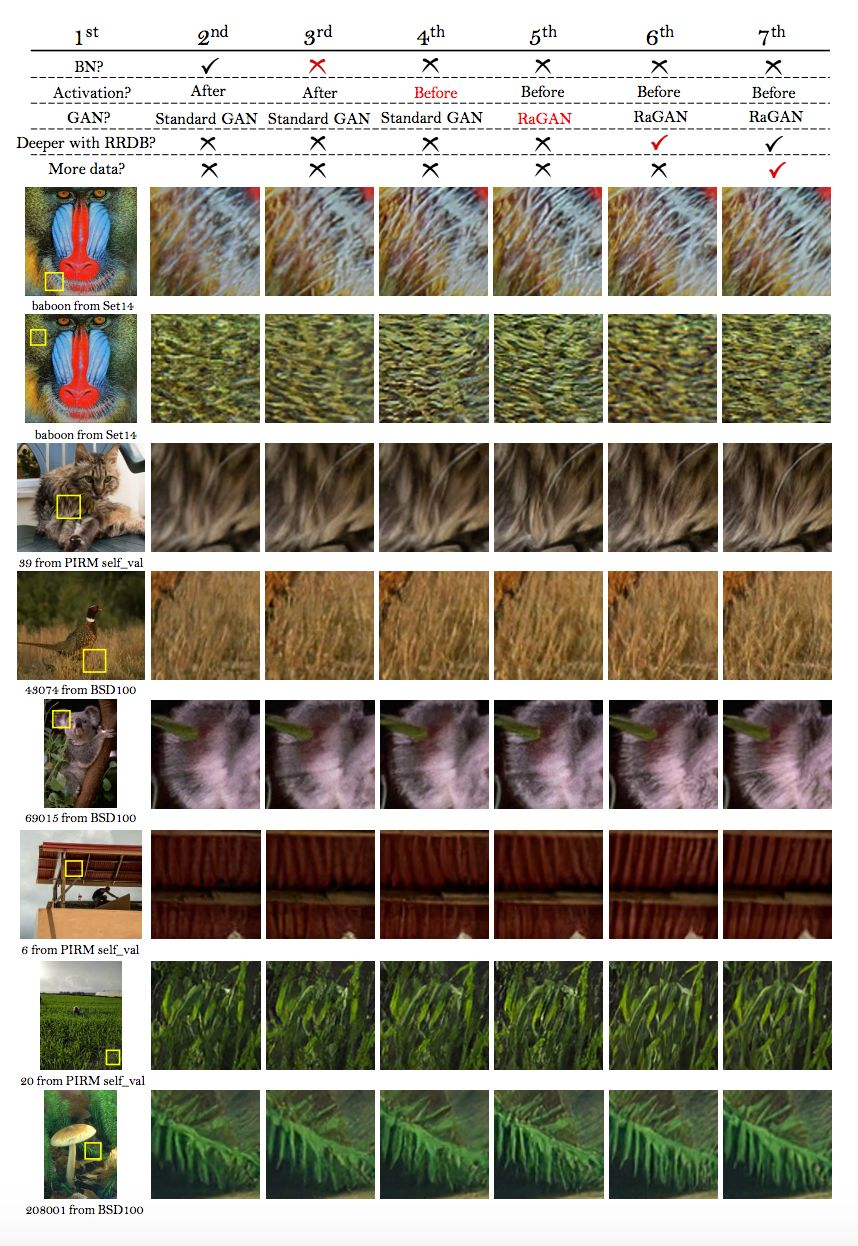1. 去掉 BN：并没有降低网络的性能，而且节省了计算资源和内存占用。而且发现当网络变深变复杂时，带 BN 层的模型更倾向于产生影响视觉效果的伪影；

2. 使用激活前的特征：得到的图像的亮度更准确，而且可以产生更尖锐的边缘和更丰富的细节；

3. RaGAN：产生更尖锐的边缘和更丰富的细节；

4. RDDB：更加提升恢复得到的纹理（因为深度模型具有强大的表示能力来捕获语义信息），而且可以去除噪声。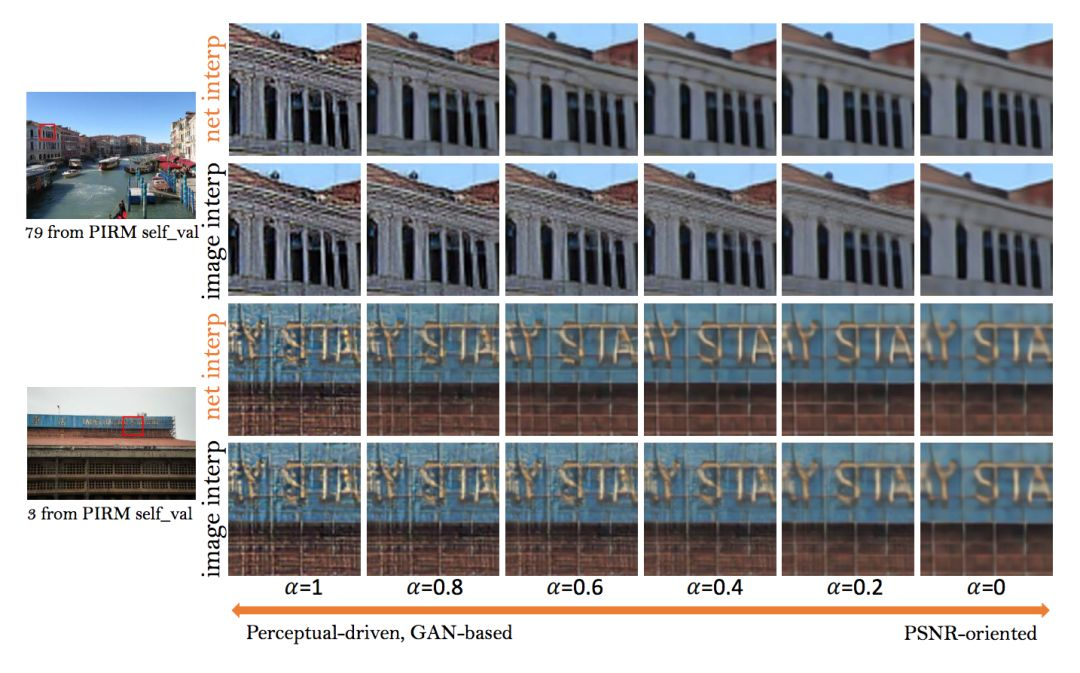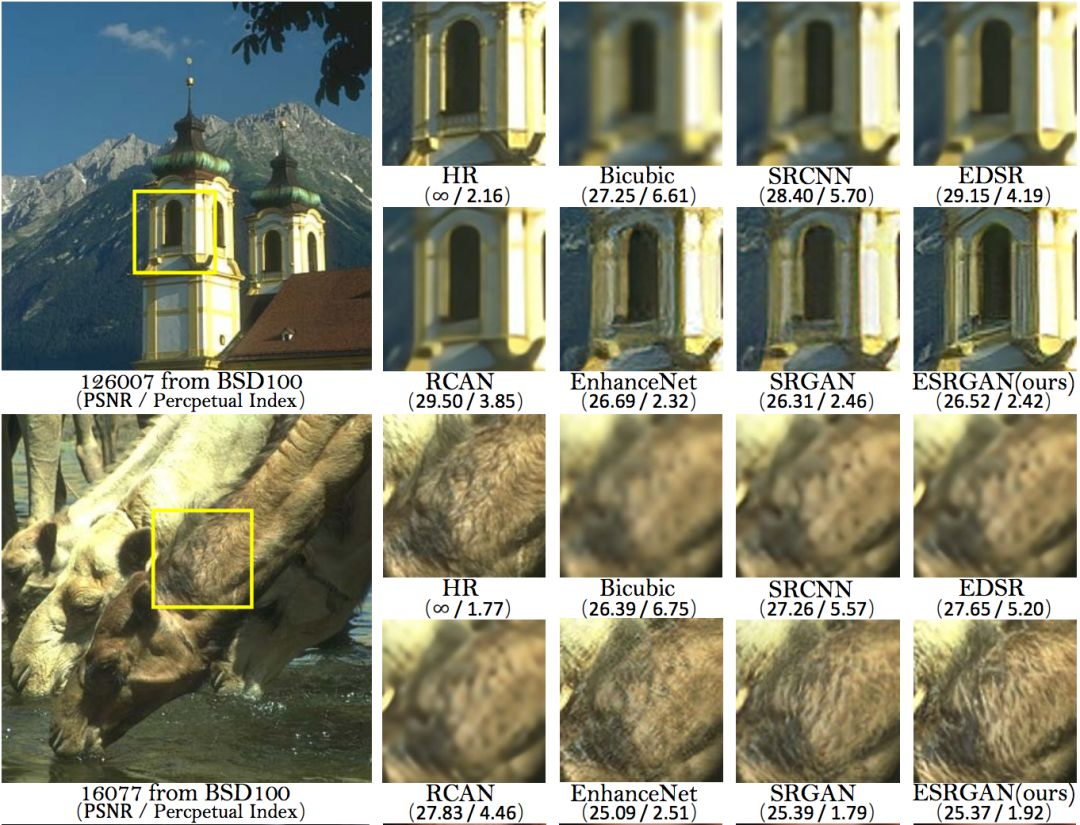### 总结PaperWeekly

VGG技术

2014年，牛津大学提出了另一种深度卷积网络VGG-Net，它相比于AlexNet有更小的卷积核和更深的层级。AlexNet前面几层用了11×11和5×5的卷积核以在图像上获取更大的感受野，而VGG采用更小的卷积核与更深的网络提升参数效率。VGG-Net 的泛化性能较好，常用于图像特征的抽取目标检测候选框生成等。VGG最大的问题就在于参数数量，VGG-19基本上是参数量最多的卷积网络架构。VGG-Net的参数主要出现在后面两个全连接层，每一层都有4096个神经元，可想而至这之间的参数会有多么庞大。

ReLU是将所有的负值都设为零，保留正值；相反，Leaky ReLU是给所有负值赋予一个非零斜率，即x＜0时，y=α·x。